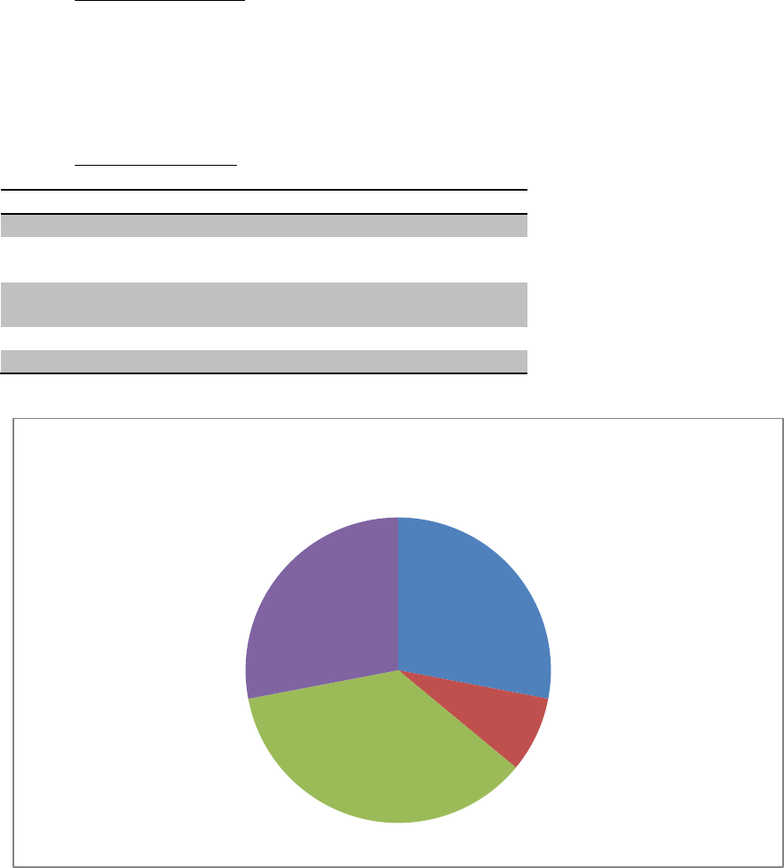Study Guides (390,000)
CA (150,000)
UTSG (10,000)
ECO (800)
all (10)
Midterm

# ECO206Y1 Study Guide - Midterm Guide: Deadweight Loss, Price Discrimination, Marginal Revenue

Department
Economics
Course Code
ECO206Y1
Professor
all
Study Guide
Midterm

This preview shows pages 1-3. to view the full 12 pages of the document.ECO206 Microeconomic Theory
1. Midterm III Structure
Based on analysis of past midterms, there are four main topics that are covered in the third midterm of
ECO206: Monopoly, Price Discrimination, Game Theory and Strategic Thinking and Oligopoly. The
midterm is usually about 2 hours long and consists of 3 to 5 questions with 3 to 5 sub-questions per
question. The total number of questions, including sub-questions, is roughly 12 to 15.
2. Midterm II Statistics
2012
2013
Monopoly
3
4
Price
Discrimination
1
1
Game Theory and
Strategic Thinking
5
4
Oligopoly
4
3
Total
13
12
Figure 1 - Midterm III Statistics
Monopoly
28%
Price
Discrimination
8%
Game Theory and
Strategic Thinking
36%
Oligopoly
28%
Midterm III Questions - Statistics

Only pages 1-3 are available for preview. Some parts have been intentionally blurred.Topic 1: Monopoly
Knowledge Summary:
Monopoly is a market structure that is defined by one seller/producer in a market
Can make decisions over how much to produce or what price to charge
The industry’s market demand curve is the same as the monopolist’s demand curve
(unlike perfect competition firm’s demand curve is perfectly elastic and industry’s
demand curve is downward sloping)
The marginal revenue of a monopolist is not equal to the price it charges because in order
to sell more output, the monopolist has to lower its prices
Monopolists are price makers whereas perfectly competitive firms are price takers
(understanding the differences between perfect competition and monopoly will help in
understanding the main fundamentals of monopoly structure).
The marginal revenue curve of a monopolist has the same intercept as the monopolist’s
demand curve, but twice the slope.
Price elasticity of demand is
greatly related to marginal revenue of
a monopolist and what prices it should
charge. If a monopolist finds itself on
an inelastic portion of the demand
curve, it can increase profits by raising
prices and lowering quantity. If it
finds itself on the elastic portion of the
demand curve it can increase profits
by lowering prices and decreasing
quantity
Keep in mind that the goal of a
monopolist, for our purposes, is to
maximize profit.
On a linear demand curve, consumer spending and thus monopolist revenue is maximized
when the price elasticity of a good is equal to -1 (i.e. it is unitary elastic). This is the point
where marginal revenue is equal to 0 (i.e. where total revenue is maximized, since marginal
revenue is the first derivative of the total revenue curve). But, recall the objective of a
monopolist is to profit maximize not revenue maximize. So it will not choose to operate
where MR=0 but instead where MR=MC (at yellow star). At this point monopolist is
producing restricted output at a higher price creates a deadweight loss. The only time
MR=0 is a profit maximizing point is if MC=0 where MC is marginal cost. Fixed costs must
be accounted for in the case where MC = 0. Examples from past tests on the next page will
elaborate on this.
At red star: MR =0, revenue is maximized at
this point and PED=-1

Only pages 1-3 are available for preview. Some parts have been intentionally blurred.Why is MC=MR the profit maximizing quantity? Because we know that: Profit = Total
Revenue Total Cost. Suppose this expression is a function of quantity (x). The first
derivatives of the RHS will be MR and MC respectively. Setting it equal to 0 we have:
MC=MR.
Useful formula for MR: MR = P(1 +
 ) where P(x) is price as a function of quantity,
and PED is price elasticity of demand.
Can also use: MR = price + (change in price)x(quantity sold at old price)
Using the first formula and letting x=1, we can say:

Rearranging we get:


, where PED is a negative number. P MC is known as the monopoly mark up, or
how much the monopolist marks up in price above the perfectly competitive price level (which is
P=MC)
And 
is the monopoly mark up ratio (or the Lerner’s index).
Summary of Questions to be Asked:
What is the profit maximizing level of output and price level?
Calculating fixed costs under positive profit levels
Illustrating monopoly profits and profit maximizing output and price level
Demand under different pricing policies (form of price discrimination, will be covered in
Topic 2 but intertwined with monopolies)
To calculate the Lerner index/ mark up ratio
Related Past Test Questions:
2013 Midterm III Question 2